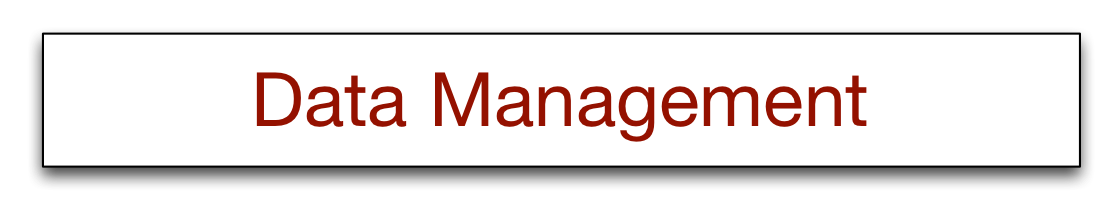# 15 - Introduction to R

1.
Access http://richardtwatson.com/data/manheim.txt which contains details of the sales of three car models: X, Y, and Z.
1a.
Compute the number of sales for each type of model.
`library(readr)url <-  'http://richardtwatson.com/data/manheim.txt't <-  read_delim(url,delim=',')t %>%  group_by(model)  %>% summarize(frequency = n())`
1c.
Report the mean price for each model.
```library(readr)library(sqldf)url <-  'http://richardtwatson.com/data/manheim.txt'
t <-  read_delim(url,delim=',')t %>%  group_by(model)  %>% summarize(mean = mean(price))```
2.
Use the 'Import Dataset' feature of RStudio to read http://richardtwatson.com/data/electricityprices.csv which contains details of electricity prices for a major city.
2a.
What is the maximum cost?
`max(electricityprices\$cost)`
2c.
What is the mean cost?
```mean(electricityprices\$cost)
```
3.
Read http://richardtwatson.com/data/electricityprices.csv containing details of the wealth of various countries and complete the following exercises.
3a.
Sort the table by GDP per capita.
`library(readr)library(sqldf)url <-  'http://people.terry.uga.edu/rwatson/data/wealth.csv't <-  read_delim(url,delim = ',')ts <-  sqldf('select * from t order by `GDP per capita`;')`
3c.
Compute the ratio of US GDP per capita to the average GDP per capita.
`library(readr)library(sqldf)url <-  'http://people.terry.uga.edu/rwatson/data/wealth.csv'sqldf('Select `GDP per capita`/(select avg(`GDP per capita`) from t) as "US ratio" from t where Country = "United States";')`
5.
Get the list of failed US banks.
5a.
Determine how many banks failed in each state.
`# download the table as csv file and read the file using RStudio's Import Datasetlibrary(sqldf)sqldf('Select ST, count(*) from banklist group by ST')`
5c.
How many banks were closed each year (hint: use the lubridate package and strptime() as sqldf does not have a YEAR function)
`library(sqldf)options(sqldf.driver = "SQLite") # to avoid a conflict with RMySQLlibrary(lubridate)# banklist\$closeYear <-  year(strptime(banklist\$Closing.Date, "%d-%b-%y"))temp <-  strptime(banklist\$Closing.Date, "%d-%b-%y")banklist\$closeYear <-  year(temp)sqldf('Select closeYear, count(*) from banklist group by closeYear;')`
6.
Get Table01.xls of U.S. broccoli data on farms and area harvested. Get rid of unwanted rows to create a spreadsheet for the area harvested with one header row and the 50 states. Change cells without integer values to 0 and save the file in CSV format for reading with R.
6a.
Reshape the data so that each observation contains state name, year, and area harvested.
```# Read the file using RStudio's Import Datase
# set the column names as R puts an X in front of the year.colnames(broccoli) <-  c('state',1974,1978,1982,1987,1992,1997,2002,2007)
library(reshape)m <-  melt(broccoli,id='state')colnames(m) <-  c('state','year','acres')   ```
6c.
Compute total hectares harvested each year for which data are available.
`library(sqldf)sqldf('select year, sum(hectares) as "Total area" from m group by year;')`